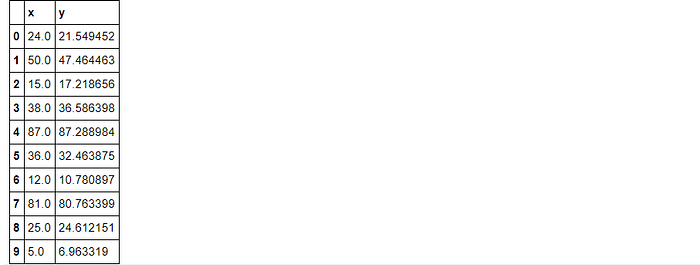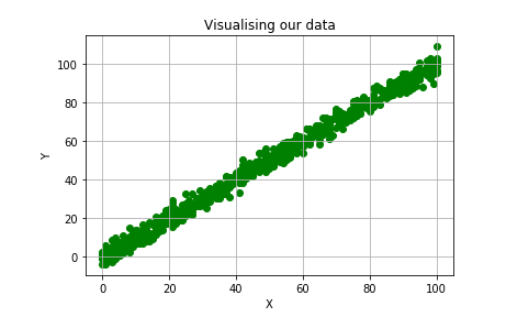Machine Learning algorithms have gained massive popularity over the last decade. Today these algorithms are used in several work fields for all sorts of data manipulation and predictions.

In this tutorial, we will be implementing the most basic machine learning algorithm called “Linear Regression”. If we were to describe linear regression in a single line, it would be something like:

“Fitting a straight line through your data points

This is a supervised machine learning algorithm. If you wish to learn about unsupervised algorithms, click here.

This tutorial will consist of 3 main parts:

· Understanding and implementing the algorithm step-by-step.

· Applying the built algorithm on 2- dimensional data (For better visualization).

· Applying the algorithm on n-dimensional data.

**The entire code used in this tutorial can be found at the following GitHub repository**.

Let’s get started.

# Step-by-step implementation

## Basic understanding

As we have discussed before, linear regression is as simple as fitting a straight line across our data, hence for basic understanding we need to revisit some basic mathematics and look at the equation for a straight line.

In the equation above, X and Y are data points and m and c are the slope and y-intercept respectively. We need the appropriate values for ‘m’ and ‘c’ in order to create an appropriate straight line.

Say we have multiple values of ‘X’ against which, we have a single ‘Y’, then we can change the above equation to create a more general one as follows:

Now instead of ‘m’ and ‘c’, now we need to find ‘c’ and all coefficients of X from a_0 to a_n and that is the complete concept of multivariable linear regression.

## Implementation

We will need the following libraries for basic math functionality and visualization.

First, we define a function that would return us predicted values of y (conventionally called Y-hat) from some given values of c and a.

Next, we need to calculate how far off we were from the original values of Y. In machine learning terms this is called calculating the cost. There are several different cost functions defined but for our case, we will use the good old “Mean-Squared Error”. The function for MSE is,

Let’s code this down:

Our aim is to minimize the cost calculated by the above function in order to come close to the original values as possible.

For this, we need to see how each of our parameters affects the overall cost. This can be done by calculating the rate of change, also known as gradient, with respect to every parameter. The equations would be:

In order to minimize the cost, we need to carry out a process called “Gradient Descent”. The graph below represents the cost with respect to a particular parameter plotted against the parameter value. In order to reach the minimum cost, we must change the value of our parameter and that is where our update equations are used.

The final equations to update the values of our parameters are as below,

Alpha in the above equation is called the learning rate. Its value needs to be optimally set, too small a value will update the parameters very slowly and too large a value can cause the updated parameters to overshoot into limbo and never converge to the global minima.

Let us code these update equations in a general fashion such that the n number of parameters can be updated.

With all the functions in place, we just need to create a training routine that would call these functions in order to minimize the cost and find suitable parameters.

### Testing

It is now time to test our algorithm. We will first test on a simpler dataset. The dataset can be downloaded for free from here. The filtering and visualizing steps of the data are carried out below.Sample Data valuesThe data seems to have a clear linear relationship so it will provide us with a good insight into how well our algorithm works.

We will normalize the data using the min-max scaling technique. Let’s define a function for min-max scaling. (There are several libraries that can do this for you but its much more fun to code)

``data_scaled = min_max_scaler(data_train)``

With normalization done, we can begin to fit data on our model.

``````X_train = data_scaled["x"].values.reshape(-1,1)
y_train = data_scaled["y"].values.reshape(-1,1)
coeff, intercept, preds = fit(X_train, y_train, iterr = 3000)``````

We run our process for 3000 iterations. Our final Loss is a significantly low value so we can say it would be a good fit. Let's visualize the results.

We can see that our model fits quite accurately on the training set and reasonably well on the test set. The results might improve if the model is trained for more iterations. (The experimentation is up to you 😀)

Another good measure to test check our model is the “R square Value” also known as “Goodness of fit”. For this purpose, we’ll use the sklearn library (because sometimes you get tired of coding😅).

``````from sklearn.metrics import r2_score
print("R_square Score:",r2_score(data_test["y"],y_pred))``````

The above score shows that a 92.6% goodness of fit is quite a good number.

### Testing on n-dimensional data

Now to check if our algorithm actually works on n-dimensional data. For this purpose, we have used the housing prices data set. (The original dataset has a lot of features but we have selected only a few of those for the purpose of this test)

Since most of the features were string types, we convert them into numerical labels.

We normalize the dataset just as before and set it to train for 2000 epochs.

``weights, biases, y_predicted = fit(X_train,y_train, iterr = 2000)``

The final cost is again quite low as we would expect. Now let's check the “R Squared Value

``````from sklearn.metrics import r2_score
print(r2_score(y_test,predicted))``````

The goodness of fit seems to be quite low, there can be multiple reasons for this; Running the algorithm for more iterations might increase the score, or as we increase the number of input parameters, the complexity of data increases so a linear relationship between the data is difficult to exist.

### Conclusion

We have successfully implemented the Linear Regression algorithm using Python and achieved a reasonably good fit on our initial data set. We did not get a very good score on our second (multi-variable) dataset because it is very difficult for such a complex dataset to follow a linear trend. For datasets like these, there are algorithms such as “Polynomial Regression” or SVM. These algorithms are a little more complex, but they achieve much better scores.

### Related Articles## SweetViz: Easy EDA and applied Data Science with Python## Understanding and Implementing Logistic Regression Algorithm (Part 2)| Python | Machine Learning## Understanding and Implementing Logistic Regression Algorithm (Part 1)| Python | Machine Learning## DBSCAN Clustering Algorithm Implementation from scratch | Python